﻿ 基于邻域系统的智能车辆最优轨迹规划方法
«上一篇文章快速检索 高级检索

 智能系统学报2019, Vol. 14Issue (5): 1040-1047  DOI: 10.11992/tis.2018050040

### 引用本文WANG Xing, ZHAO Hailiang, WANG Zhigang. Optimal trajectory planning method of intelligent vehicles based on neighborhood system[J]. CAAI Transactions on Intelligent Systems, 2019, 14(5): 1040-1047. DOI: 10.11992/tis.201805004.### 文章历史

Optimal trajectory planning method of intelligent vehicles based on neighborhood system
WANG Xing, ZHAO Hailiang , WANG Zhigang
Department of Information and Computation Science, School of Mathematics, Southwest Jiaotong University, Chengdu 610031, China
Abstract: This study mainly investigates the trajectory planning and control problems in the driving process of smart vehicles. First, based on the theory of neighborhood system, the dynamic control of intelligent vehicles on complex roads is transformed into a simple static control in the neighborhood, and then the optimal trajectory is selected in the neighborhood. The bending resistance index of the curve is defined by the integral of the curvature, and based on this, the optimal trajectory curve evaluation model and algorithm in the neighborhood is given. Finally, a satisfactory trajectory curve established by the interpolation method is taken as an example for simulation. The results show that this method has some advantages in selecting the steadiness and smoothness of the driving trajectory of an intelligent vehicle.
Key words: intelligent vehicle    automatic driving    trajectory planning    obstacle avoidance    neighborhood system    optimal trajectory    satisfaction curve    comprehensive evaluation

1 智能车辆的邻域系统

1)根据控制过程中观测变量的特点选择合适的邻域系统；

2)通过一些优化方法，在当前状态下确定一个满意的可行邻域；

3)在满意的邻域中，根据一些规划方法给予满意的决策，实施新的决策行为；

4)在可行邻域的持续时间内保持相同的行为，直到产生下一个决策；

5)上述过程是一个完整的决策循环。当一个周期完成后，一个新的循环开始依次执行步骤2)～4)。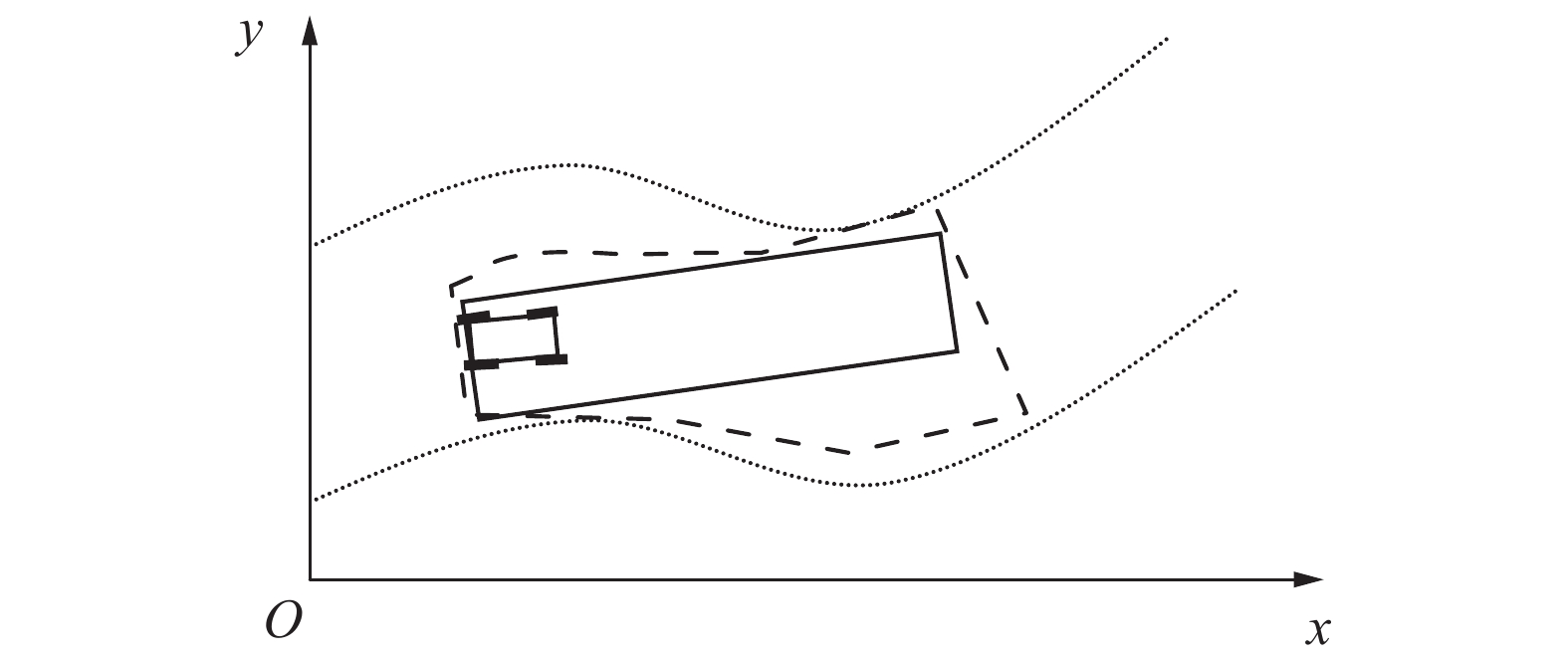Download: 图 1 智能车标准可行邻域分析图 Fig. 1 Standard feasible neighborhood analysis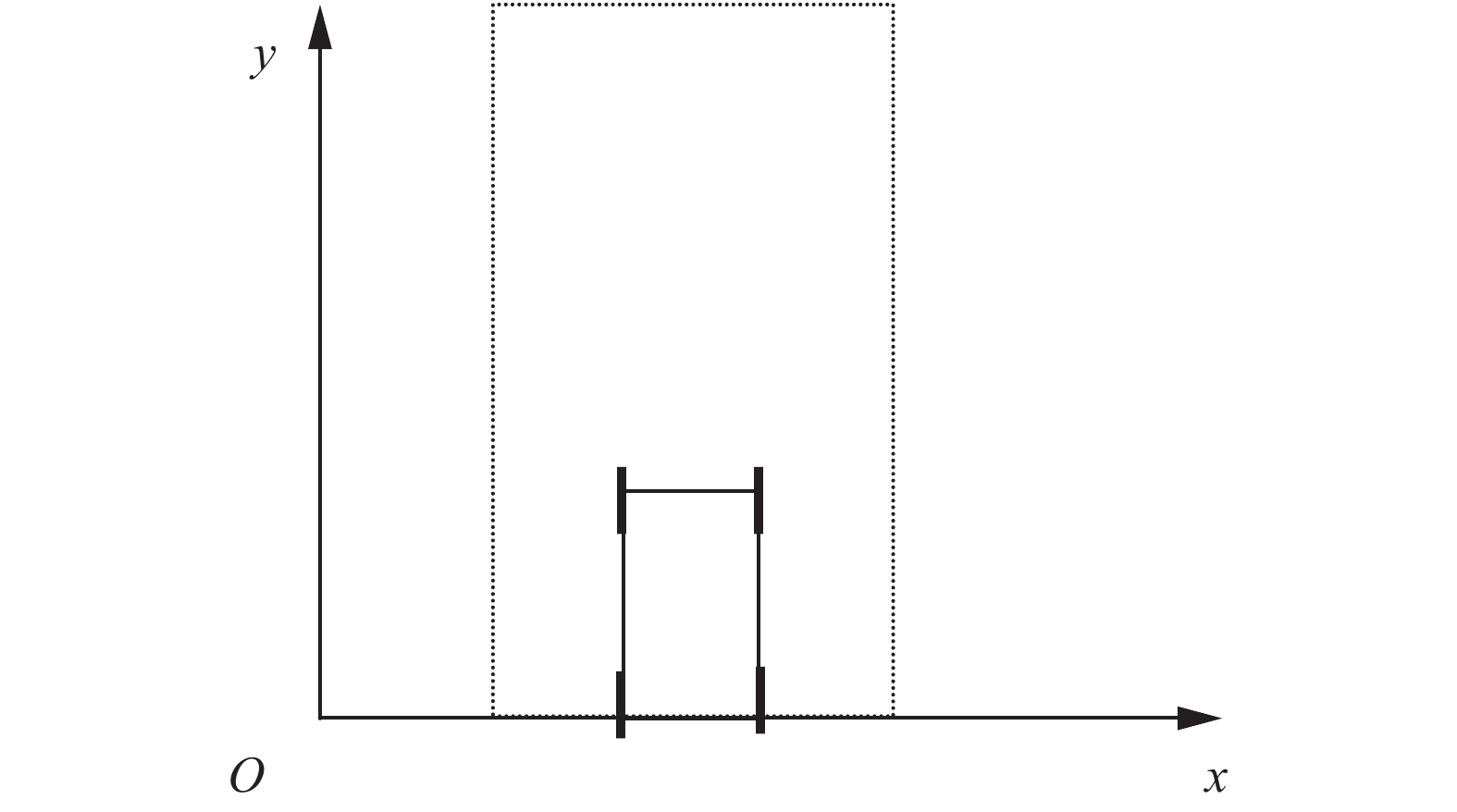Download: 图 2 标准矩形邻域 Fig. 2 Standard rectangular neighborhood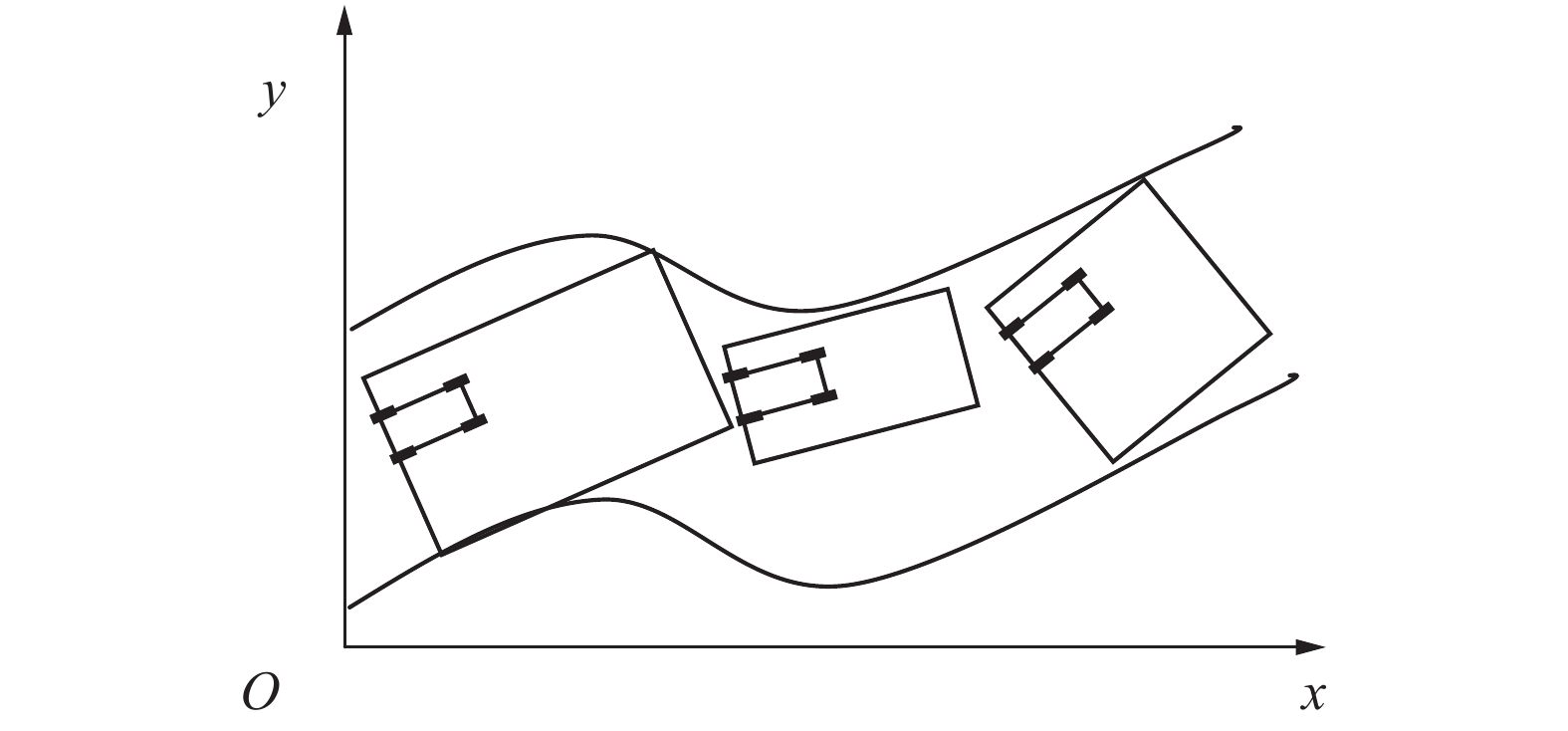Download: 图 3 智能车的动态轨迹规划 Fig. 3 Intelligent vehicles dynamic trajectory planning
2 最优轨迹曲线的评判指标 2.1 基于邻域系统的最优轨迹曲线

 $J\left[ {x\left( t \right)} \right] = \int_0^T {F\left( {t,x\left( t \right),x'\left( t \right)} \right){\rm{d}}t}$ (1)

1)用时较短；

2)行走路径较短；

3)乘客感到舒适。

2.2 最优轨迹曲线的评判指标

1)轨迹曲线的首尾位置是固定的，起始位置为当前邻域相对坐标系的原点，末位置为当前标准可行邻域的尾部中点向左平移一个车体长度。

2)轨迹曲线的方程为单值函数，即对于自变量x，其对应的函数值f(x)是唯一的。

3)轨迹曲线是连续的。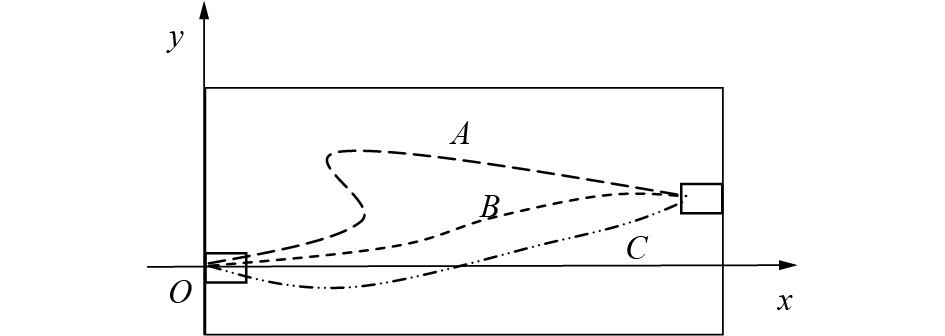Download: 图 4 曲线构造图 Fig. 4 Curve construction diagram

 $J = J\left[ {y\left(x \right)} \right]{\rm{ = }}\int_{{x_0}}^{{x_1}} {\sqrt {1{\rm{ + }}{{\left( {y'\left( x \right)} \right)}^2}} } {\rm{d}}x$ (2)

 $K = K\left[ {y\left( x \right)} \right] = \int_{{x_0}}^{{x_1}} {\frac{{\left| {y''\left( x \right)} \right|}}{{\sqrt {{{\left( {1{\rm{ + }}y'\left( x \right)} \right)}^3}} }}} {\rm{d}}x$ (3)

2.3 评判指标的标准化

 $J^* = 1{\rm{ - }}\displaystyle\frac{{\displaystyle\frac{{\int_{{x_0}}^{{x_1}} {\sqrt {1{\rm{ + }}{{\left( {y'\left( x \right)} \right)}^2}} } dx}}{{{\rm{1 + }}\int_{{x_0}}^{{x_1}} {\sqrt {1{\rm{ + }}{{\left( {y'\left( x \right)} \right)}^2}} } dx}} - \displaystyle\frac{{\left( {{x_1} - {x_0}} \right)}}{{{\rm{1 + }}\left( {{x_1} - {x_0}} \right)}}}}{{1 - \displaystyle\frac{{\left( {{x_1} - {x_0}} \right)}}{{{\rm{1 + }}\left( {{x_1} - {x_0}} \right)}}}}$ (4)

 $J_{\min }^*{\rm{ = }}\frac{{\left( {{x_1} - {x_0}} \right)}}{{{\rm{1 + }}\left( {{x_1} - {x_0}} \right)}}$ (5)

 $J^*=1{\rm{ - }}\frac{{\frac{J}{{{\rm{1 + }}J}} - J_{\min }^*}}{{1 - J_{\min }^*}} = \frac{1}{{\left( {{\rm{1 + }}J} \right)\left( {1 - J_{\min }^*} \right)}}$ (6)

 $K^* = 1{\rm{ - }}\displaystyle\frac{{\int_{{x_0}}^{{x_1}} {\displaystyle\frac{{\left| {y''\left( x \right)} \right|}}{{\sqrt {{{\left( {1{\rm{ + }}y'\left( x \right)} \right)}^3}} }}} {\rm{d}}x}}{{1{\rm{ + }}\int_{{x_0}}^{{x_1}} {\displaystyle\frac{{\left| {y''\left( x \right)} \right|}}{{\sqrt {{{\left( {1{\rm{ + }}y'\left( x \right)} \right)}^3}} }}} {\rm{d}}x}}$ (7)

 $K^* = 1{\rm{ - }}\frac{K}{{1 + K}} = \frac{1}{{1 + K}}$ (8)

 $C^* = {w_1} \cdot J^*{\rm{ + }}{w_2} \cdot K^*$ (9)

3 最优轨迹曲线模型及其求解方法 3.1 最优轨迹曲线模型及其满意条件

 $\left\{ \begin{array}{l} \min T \\ \min J\left[ {y\left( x \right)} \right] \\ \min K\left[ {y\left( x \right)} \right] \\ \end{array} \right.$ (10)

 $\left\{ \begin{array}{l} y\left( {{x_0}} \right) \subset \varGamma \left( {{x_0}} \right) \subset Q \\ T \leqslant {T_{\max }} \\ \end{array} \right.$ (11)

 $B = \left\{ {y\left( x \right){\rm{|}}{w_1} \cdot J^*\left[ {y\left( x \right)} \right]{\rm{ + }}{w_2} \cdot K^*\left[ {y\left( x \right)} \right] \geqslant p} \right\}$ (12)

3.2 满意曲线的求解算法

1)确定初始值。

 $\left\{ {\begin{array}{*{20}{l}} {y\left( {{x_1}} \right){\rm{ = }}0,}&{{x_1}{\rm{ = }}0}\\ {y\left( {{x_2}} \right){\rm{ = }}{y_2},}&{{x_1} < {x_2} < {x_3}}\\ {y\left( {{x_3}} \right) = s,}&{{x_3}{\rm{ = }}H - l} \end{array}} \right.$ (13)

 $\left\{ \begin{array}{l} y'\left( {{x_1}} \right){\rm{ = }}0\\ y'\left( {{x_2}} \right) = y_2'\\ y'\left( {{x_3}} \right){\rm{ = }}0 \end{array} \right.$ (14)

 ${k_0} = s/h - l$ (15)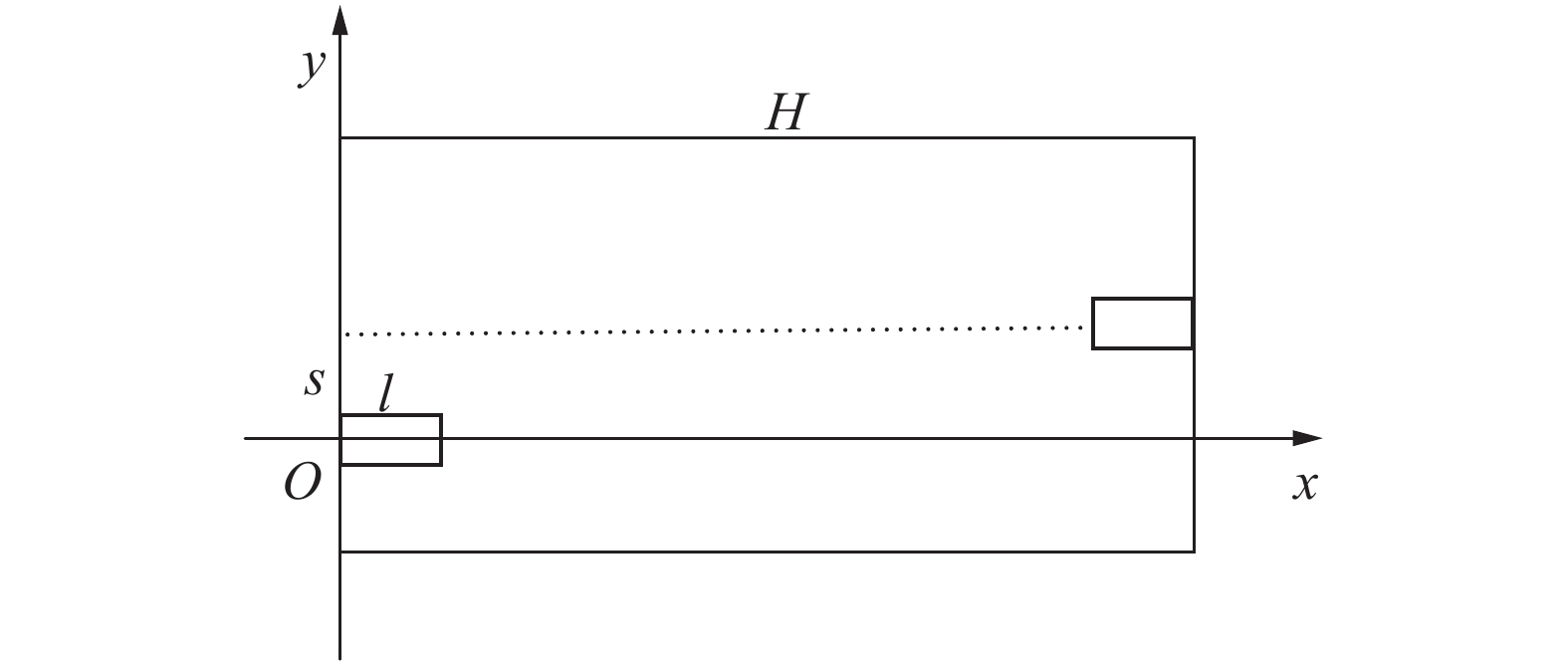Download: 图 5 智能车的邻域坐标系 Fig. 5 Neighborhood of intelligent vehicles coordinate system

2)计算轨迹曲线指标。

 ${H_{2n + 1}}\left( x \right){\rm{ = }}\sum\limits_{i = 1}^n {{H_i}\left( x \right)} \cdot {y_i}{\rm{ + }}\sum\limits_{k = 1}^n {{h_k}\left( x \right)} \cdot {y_k}'$ (16)

3)计算导数值。

 ${k_i} = \left( {\alpha + ti} \right){k_0}$ (17)

1)节点坐标为 $\left( {\displaystyle\frac{{H - l}}{2},\frac{s}{2}} \right)$ ，为曲线的中点。

2)节点一阶导数为 $\displaystyle\frac{{3s}}{{2\left( {H{\rm{ - l}}} \right)}}$

3.3 满意曲线的理论分析

 $\zeta = \sup C^*\left[ {y\left( x \right)} \right]$ (18)

 $C_{1}^* \leqslant C_{2}^* \leqslant \cdots \leqslant C_{{i - 1}}^* \leqslant C_{i}^*$ (19)

 $\mathop {\lim}\limits_{i \to \infty } {\rm{ }}C_{i}^* = \zeta$ (20)

4 仿真结果以及同其他文献的对比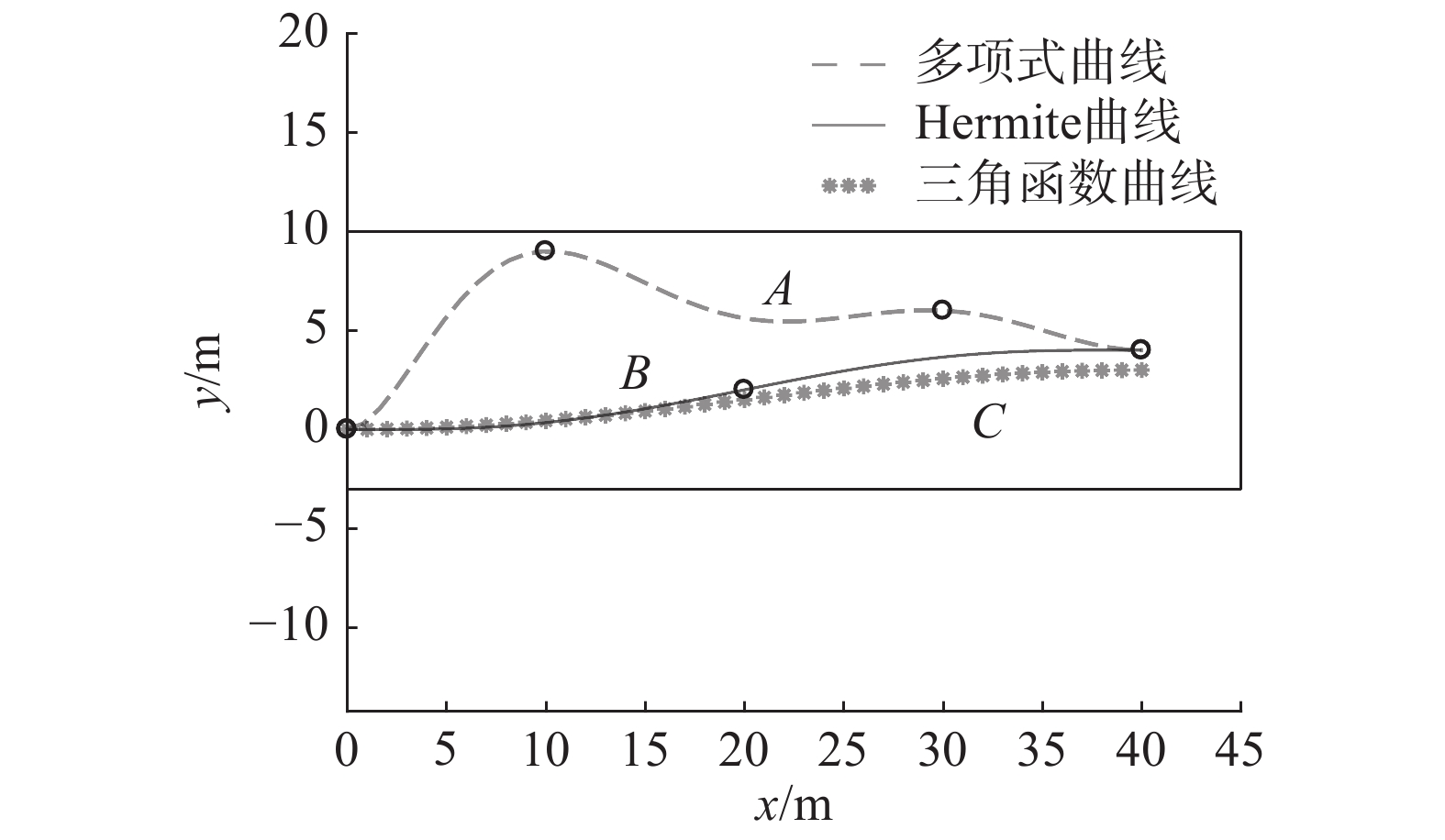Download: 图 6 实例对比图 Fig. 6 Example comparison chart

 $\begin{array}{l} {y_{\rm{A}}}\left( x \right){\rm{ = }} - 1.21 \cdot {10^{ - 8}} \cdot {x^7} + 2.08 \cdot {10^{ - 6}} \cdot {x^6} - 1.40 \cdot {10^{ - 4}} \cdot {x^5} {\rm{ + }} \\ \qquad\qquad 4.65 \cdot {10^{ - 3}} \cdot {x^4} - 7.63 \cdot {10^{ - 2}} \cdot {x^3} + 5.09 \cdot {10^{ - 1}} \cdot {x^2} \\ \end{array}$
 $\begin{array}{l} {y_{\rm{B}}}\left( x \right){\rm{ = }} 3.13 \cdot {10^{ - 7}} \cdot {x^5} - 3.13 \cdot {10^{ - 5}} \cdot {x^4} + {\rm{ }} \\\quad\qquad 8.75 \cdot {10^{ - 4}} \cdot {x^3} - 2.50 \cdot {10^{ - 3}} \cdot {x^2} \\ \end{array}$
 ${y_{\rm{C}}}\left( x \right){\rm{ = }}\frac{3}{2} \cdot \sin \left( {\frac{{{\text{π}} \cdot x}}{{40}} - \frac{\pi }{2}} \right) + \frac{3}{2}$

 $J\left[ {{y_A}\left( x \right)} \right] = 44.832\text{、}J\left[ {{y_B}\left( x \right)} \right] = 40.304\text{、}J\left[ {{y_C}\left( x \right)} \right] = 40.138$

 $K\left[y_{A}(x)\right]\!=\!333\;18\text{、}\!K\left[y_{B}(x)\right]\!=\!0.402\;2\text{、}\!K\left[y_{C}(x)\right]\!=\!0.993\;1$

 $J^{*}\left[y_{A}(x)\right]=0.903\text{、}J^{*}\left[y_{B}(x)\right]=0.992\text{、}J^{*}\left[y_{C}(x)\right]=0.996$
 $K^{*}\left[y_{A}(x)\right]=0.231\text{、}K^{*}\left[y_{B}(x)\right]=0.713\text{、}K^{*}\left[y_{C}(x)\right]=0.501$

 $C^*\left[y_{A}(x)\right]=0.433\text{、}C^*\left[y_{B}(x)\right]=0.797\text{、}C^{*}\left[y_{C}(x)\right]=0.649$

 $C^{*}\left[y_{A}(x)\right]=0.499\text{、}C^{*}\left[y_{B}(x)\right]=0.824\text{、}C^{*}\left[y_{C}(x)\right]=0.699$

 $C^{*}\left[y_{A}(x)\right]=0.533\text{、}C^{*}\left[y_{B}(x)\right]=0.838\text{、}C^{*}\left[y_{C}(x)\right]=0.723$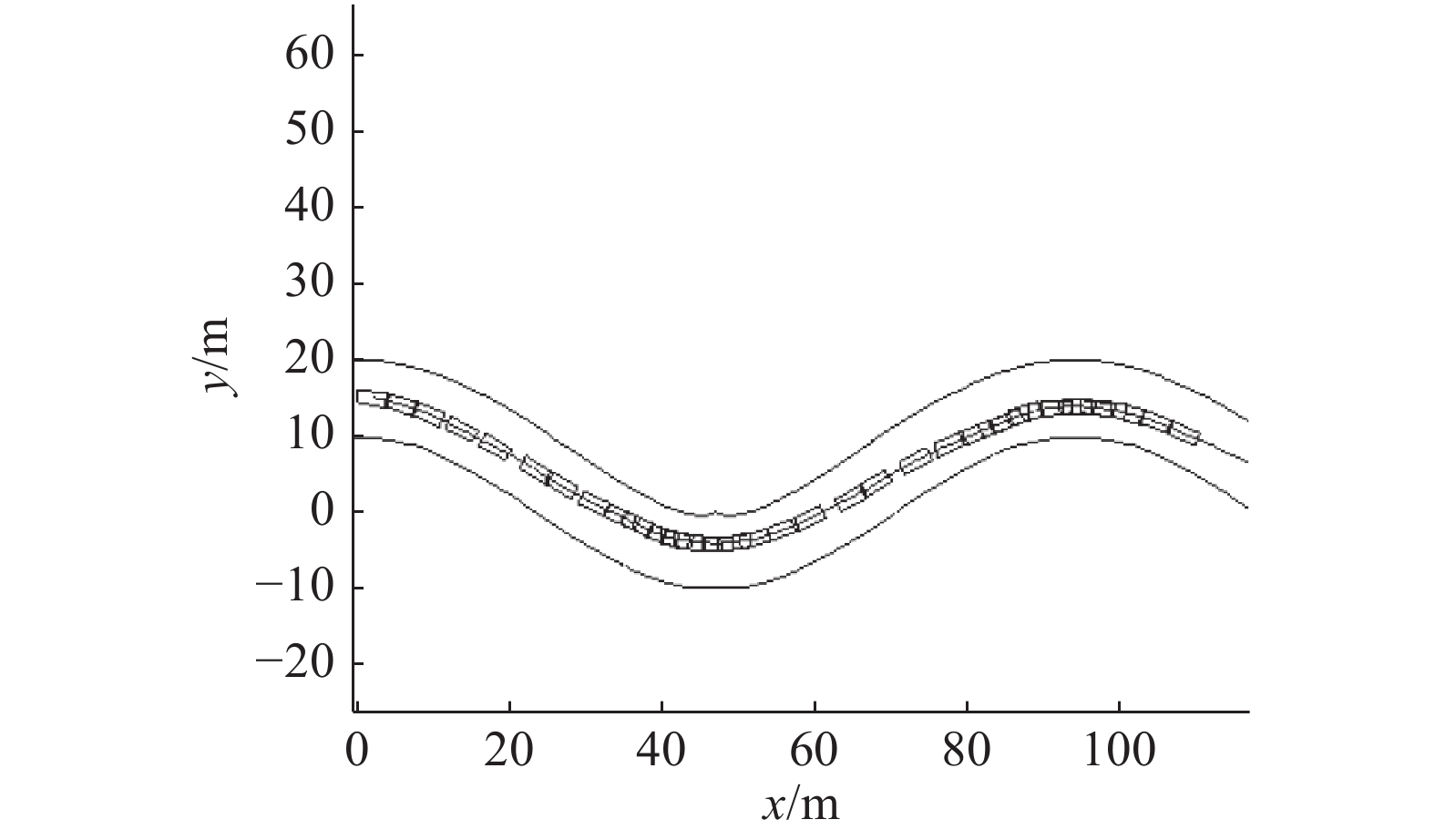Download: 图 7 道路仿真图 Fig. 7 Road simulation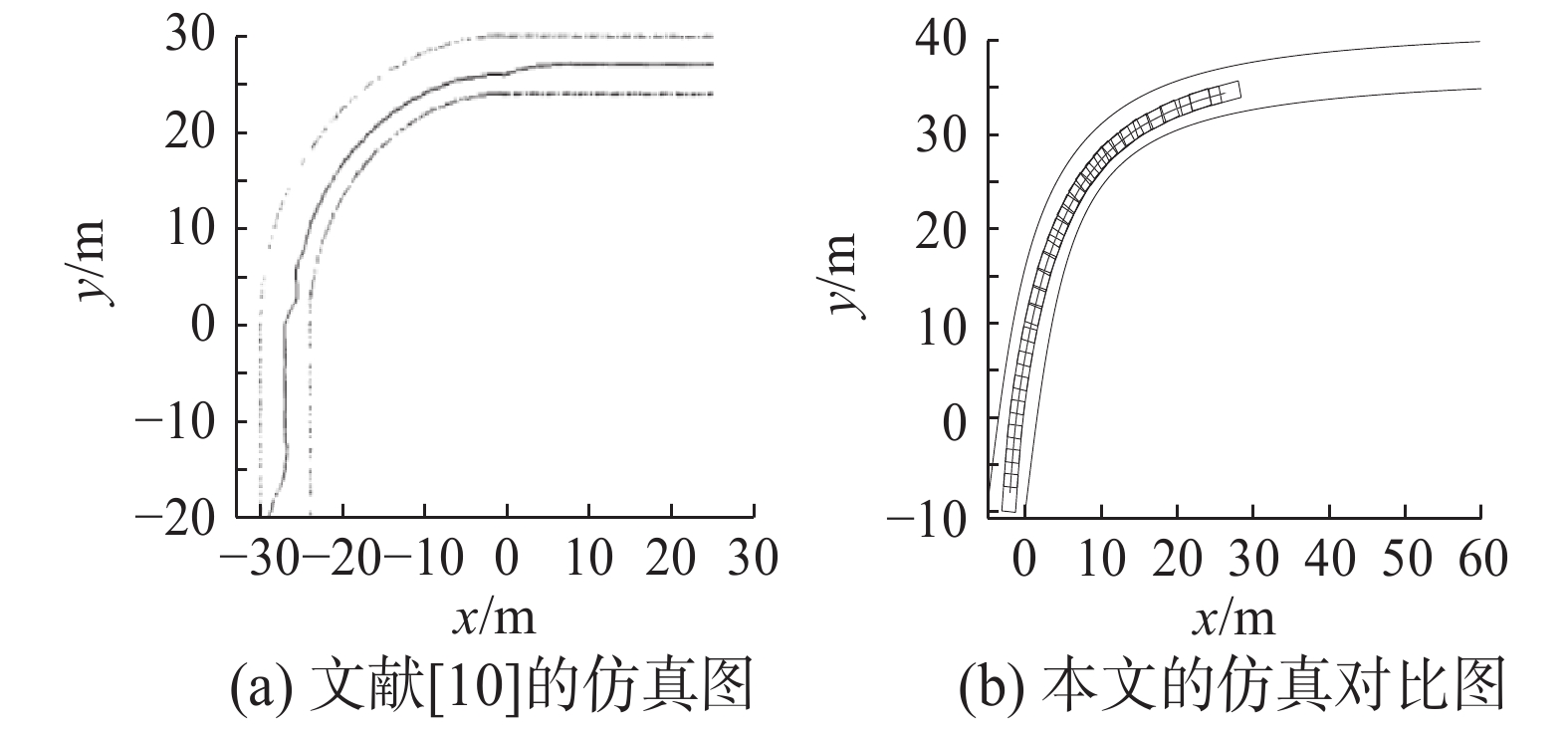Download: 图 8 文献与本文仿真图对比 Fig. 8 Comparison of the literature  with the simulation of this paper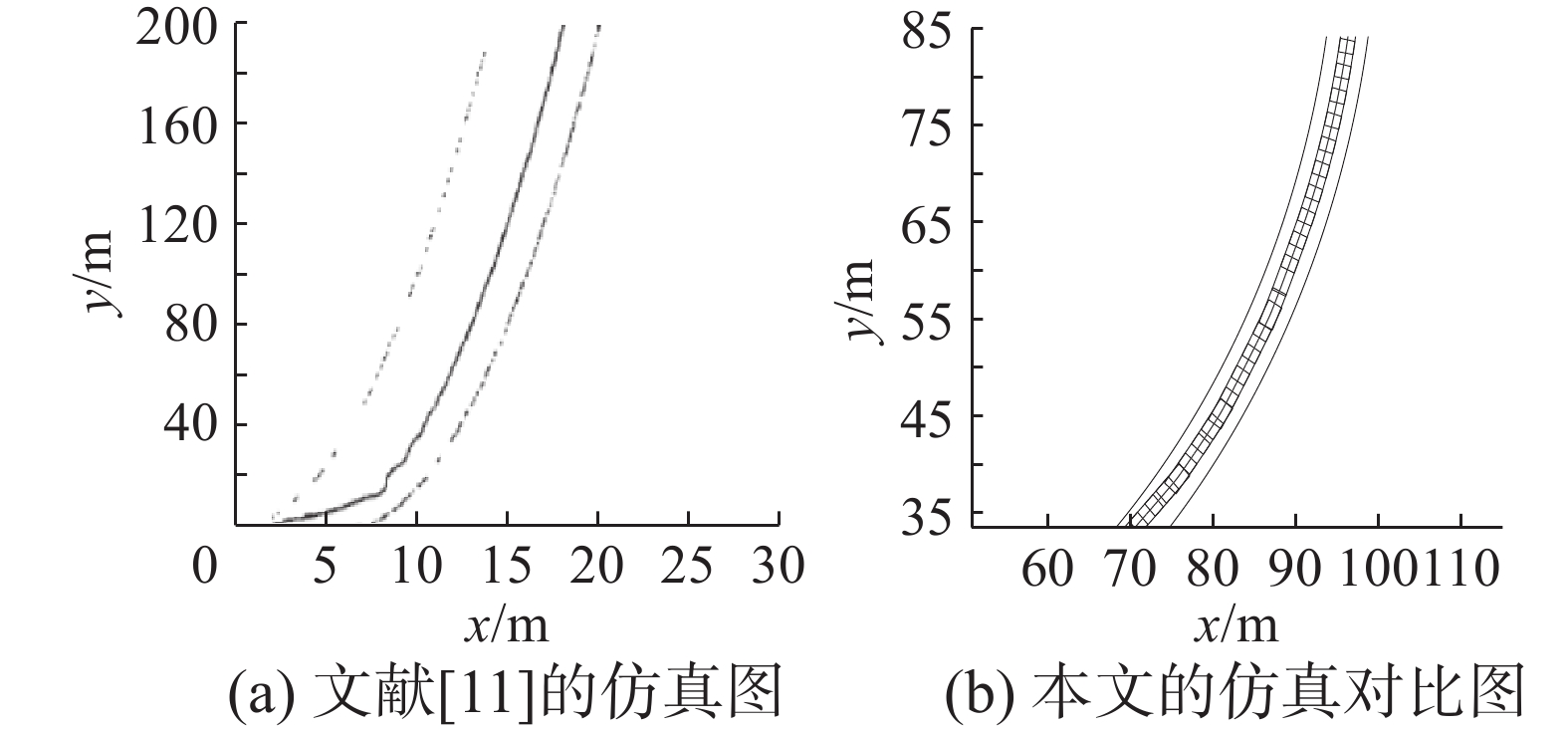Download: 图 9 文献与本文仿真图对比 Fig. 9 Comparison of the literature  with the simulation of this paper
5 结束语

  ZHANG Kailong, YANG Ansheng, SU Hang, et al. Unified modeling and design of reservation-based cooperation mechanisms for intelligent vehicles[C]//Proceedings of the IEEE 19th International Conference on Intelligent Transportation Systems. Rio de Janeiro, Brazil, 2016: 1192–1199. (0)  ZHANG Kailong, ZHANG Dafang, DE LA FORTELLE A, et al. State-driven priority scheduling mechanisms for driverless vehicles approaching intersections[J]. IEEE transactions on intelligent transportation systems, 2015, 16(5): 2487–2500. (0)  ZHANG Ronghui, YOU Feng, CHEN Fang, et al. Vehicle detection method for intelligent vehicle at night time based on video and laser information[J]. International journal of pattern recognition and artificial intelligence, 2018, 32(4): 1850009. DOI:10.1142/S021800141850009X (0)  JONES S, HUSS A, KURAL E, et al. Optimal electric vehicle energy efficiency & recovery in an intelligent transportation system[C]//Proceedings of the 19th ITS World Congress. Vienna, Austria, 2012: 132–133. (0)  叶炜垚. 基于虚拟障碍物的智能车导航方法研究[D]. 上海: 上海交通大学, 2011. YE Weiyao. Virtual obstacles based intellgent vehicle navigatton method research[D]. Shanghai: Shanghai Jiaotong University, 2011. (0)  YAO Peng, WANG Honglun, SU Zikang. Cooperative path planning with applications to target tracking and obstacle avoidance for multi-UAVs[J]. Aerospace science and technology, 2016, 54: 10-22. DOI:10.1016/j.ast.2016.04.002 (0)  游峰. 智能车辆自动换道与自动超车控制方法的研究[D]. 长春: 吉林大学, 2005. YOU Feng. Study on autonomous lane changing and autonomous overtaking control method of intelligent vehicle[D]. Changchun: Jilin University, 2005. (0)  ZHAO Hailiang. A dynamic optimization decision and control model based on neighborhood systems[C]//Proceedings of the 2013 6th International Congress on Image and Signal Processing. Hangzhou, China, 2013: 1319–1324. (0)  付慧敏. 基于邻域系统和软优化的智能车自动倒车控制算法[D]. 成都: 西南交通大学, 2016. FU Huimin. Reverse parking control method based on neighborhood system and soft optimization[D]. Chengdu: Southwest Jiaotong University, 2016. (0)  刘恒. 模糊多目标优化控制在汽车控制中的应用[D]. 成都: 西南交通大学, 2006. LIU Heng. The study of Multi-object optimal control on application in vehicle control[D]. Chengdu: Southwest Jiaotong University, 2006. (0)  徐永. 多目标优化模糊控制及在车辆自动驾驶中的应用[D]. 成都: 西南交通大学, 2008. XU Yong. Optimization of Multi-objective fuzzy control and application in vehicle control[D]. Chengdu: Southwest Jiaotong University, 2008. (0)  江怡. 基于粗糙集和邻域系统的智能车避障控制方法[D]. 成都: 西南交通大学, 2017. JIANG Yi. A control method to avoid obstacles for an intelligent car based on rough sets and neighborhood system[D]. Chengdu: Southwest Jiaotong University, 2017. (0)